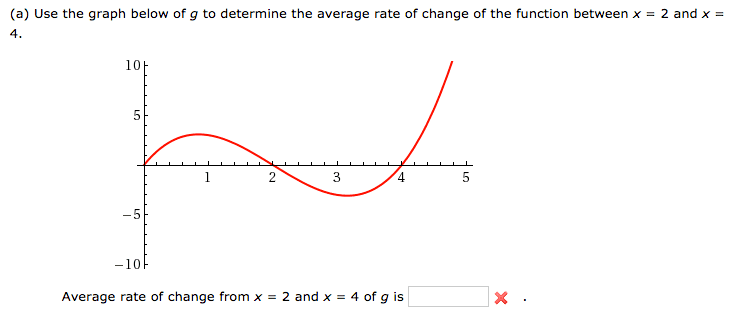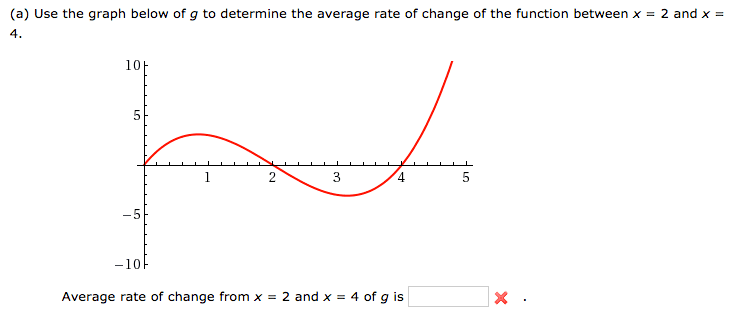# Precalculus -- determine the rate of change of the function

## Homework Statement## Homework Equations

Rate of change formula: y2-y1/x2-y2

## The Attempt at a Solution

If this is a rate of change problem so I use the rate of change formula: y2-y1/x2-y2
and since it's from 2 to 4 I think that would be (2,0) (4,0)
and thus (0-0)/(4-2) = 0/2 = 0

I don't think this is right. What am I doing wrong?

Mark44
Mentor

## Homework Statement## Homework Equations

Rate of change formula: y2-y1/x2-y2

## The Attempt at a Solution

If this is a rate of change problem so I use the rate of change formula: y2-y1/x2-y2
and since it's from 2 to 4 I think that would be (2,0) (4,0)
and thus (0-0)/(4-2) = 0/2 = 0

I don't think this is right. What am I doing wrong?